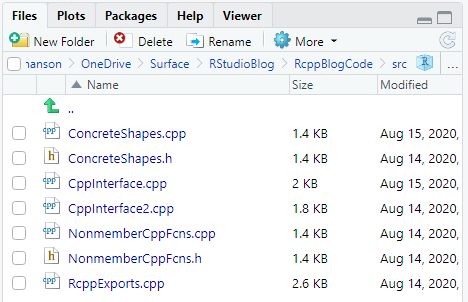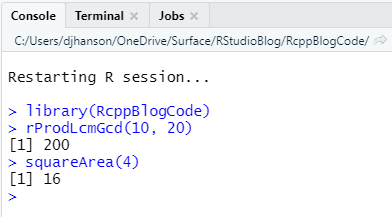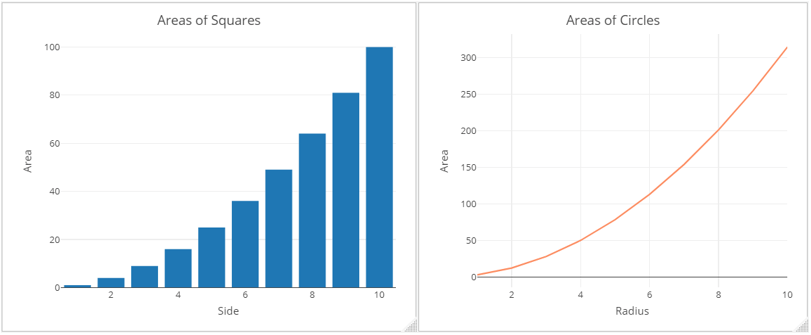Want to share your content on R-bloggers? click here if you have a blog, or here if you don't.

Daniel Hanson is a full-time lecturer in the Computational Finance & Risk Management program within the Department of Applied Mathematics at the University of Washington.

In the previous post, we went through the build processes for a simple package containing a single C++ `.cpp` file, using the `rcpp_hello_world.cpp` example that is included by default when one creates a new R package using `Rcpp` in RStudio.

What we’ll do now is examine a more realistic case which involves multiple reusable C++ files as well as the C++ interface files that export functions to R. For this example, you should download the C++ files from this GitHub repository. In addition, you should download a set of test functions in R, here (RStudioBlogTests.R). Please note that this file is not part of the package code; we will discuss this shortly.

## Building an `Rcpp` Package with Reusable C++

The code you have downloaded from the first link is the same as that referenced in Part 3 of this series. You will now create an `Rcpp` package project in RStudio and import this code.

### Create an RStudio Project

This procedure is the same as what we covered in the previous post. For convenience later, I suggest you name your project `RcppBlogCode`. Make sure to modify the `NAMESPACE` file as we discussed before. Also, while not mandatory, you may find it simpler to delete the `rcpp_hello_world.cpp` file; we will not use it in this example.

### Import the C++ Code into the Project

Next, copy or move the code you downloaded from the `/src` subdirectory on GitHub into the `/src` subdirectory of your `RStudio` project. When this is done, you should see the files present in this location in the files pane in RStudio:Note that this source code contains:

• Declaration (header files) for the reusable C++ code

• `NonmemberCppFcns.h`: Declarations of nonmember functions
• `ConcreteShapes.h`: Declarations of the `Square` and `Circle` classes
• Files containing implementations of functions and classes in the reusable C++ code

• `NonmemberCppFcns.cpp`: Nonmember function implementations
• `ConcreteShapes.cpp`: `Square` and `Circle` class implementations
• C++ files that call reusable code and export functions to R (`.cpp` files only); each exported function is indicated by the `//` `[[Rcpp::export]]` tag

• `CppInterface.cpp`: Calls nonmember functions in the code base
• `CppInterface2.cpp`: Creates and uses instances of classes in the code base

### Build and Run Functions in the R Package

Next, let’s build this package, just as we did for our simpler example in the previous post. Select `Clean and Rebuild` from the `Build` menu at the top of the RStudio IDE. When complete, R will restart, and the `RcppBlogCode` package will be loaded into your R session. You can now call functions from this package in the console; e.g. ,

• Calculate the product of the LCM and GCD of 10 and 20: `rProdLcmGcd(10, 20)`
• Calculate the area of a square with length 4: `squareArea(4)`Try package functions in console

Congratulations again! You have now successfully imported resuable C++ code, independent of R and `Rcpp`, and used it via an interface as an R function in your generated package. However, this of course is not a realistic scenario. What we really want is to use this package in a regular and independent R session.

## Use the Package Independently of the `Rcpp` Project

So far, you have built an R package containing C++ code, and you have called a couple of functions from the console, but that of course is not what a real R user does. What you’ll want to do is open a new (and empty) RStudio instance. As the `RcppBlogCode` package is installed on your machine, all you need to do is load it as you would any other R package:

`library(RcppBlogCode)`

Next, open up the `RStudioBlogTests.R` test file that you downloaded at the outset. Again, make sure this file is located outside of the `Rcpp` package directory structure. You can now use the package functions that call the C++ nonmember functions internally in the package:

```rAdd(531, 9226)

(x <- c(5:1))
rSortVec(x)

rProdLcmGcd(10, 20)
```

You can also run the R functions that create instances of the `Square` and `Circle` classes in the C++ code:

```squareArea(4)
circleArea(1)```

The actual mathematics here are obviously nothing exciting, but you should now see that you have a blueprint that takes in independent and reusable C++ source code, wraps its functionality in C++ interface functions that get exported by `Rcpp`, and allows you to use these exported functions just as you would any other R package functions.

Perhaps more interesting is to see how you can take the results of these package functions and use them in other R functions and packages. For this next example, you will need to install and load the `plotly` package. We will define vectors of square sides and circle radii, and compute the corresponding areas using our package functions. This is again nothing earth-shattering, but we can then use them in `plotly` to generate some reasonably professional quality graphs:

```library(plotly)

squareSides <- c(1:10)

squareAreas <- sapply(squareSides, squareArea)

### Use plotly to visualize some results:
dfSq <- matrix(data=c(squareSides, squareAreas), nrow=length(squareSides), ncol=2)
dfSq <- as.data.frame(dfSq)
colnames(dfSq) = c("Side", "Area")
rownames(dfSq) = NULL
plot_ly(dfSq, x = ~Side, y = ~ Area, type = "bar") %>%
layout(title="Areas of Squares")

dfCirc <- matrix(data=c(circleRadii, circleAreas), nrow=length(squareSides), ncol=2)
dfCirc <- as.data.frame(dfCirc)
rownames(dfCirc) = NULL
plot_ly(dfCirc, x = ~Radius, y = ~ Area, type = 'scatter',
mode = 'lines', color = 'red') %>%
layout(title="Areas of Circles")
```Using results from package in Plotly graphs

Remark 1: If you wish to go back and modify the package code, you will need to close your R session that is using the package; otherwise, the code will not build.

Remark 2: As discussed last time, you can also export the package to a binary and deploy it on another machine. In general, this allows you to design and build your own `Rcpp` R package, and deploy it for your colleagues to use, if you wish.

## Summary

You have now seen how to import standard and reusable C++ code into an R package using `Rcpp`, use the package in R, and deploy it as a binary. The one remaining topic to cover is documentation, which we will take up next time.